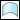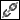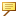Surfacing > Creo Interactive Surface Design (Style) > Curves > Editing Curves > About Unlinking and Converting Curves
Curves in Style can have references to other geometric entities. Some of the references are required, while others are optional. For example, a planar curve must have a plane reference or it cannot continue to be a curve of the planar type. However, soft point references and tangent constraints are optional, as you can remove them without changing the type of the curve. For surfaces, connections and internal curves are optional references.
Use theStyle >Unlink andStyle > Curve > Convert commands to manage references.
Unlink removes all optional references, but does not change the type of the curve. Unlink is applicable to curves defined by points, that is, free, curve on surface (COS), and planar curves. However, Unlink is not applicable to a dropped COS or a COS by intersect.
Convert changes the type of the curve. Convert is applicable to COS defined by points, dropped COS, and COS by intersect.
When a curve is a COS defined by points, Convert converts it to a free curve.
When a curve is a dropped COS:
Curve is dropped onto a datum plane—Convert converts the curve to a planar curve.
Curve is dropped onto a surface, intent surface, or quilt—Convert converts the curve to a COS defined by points.
When a curve is a COS by intersect, Convert converts it to a COS defined by points.• Use Convert twice on a dropped COS or a COS by intersect to convert the curve to a free curve.• COSs created by drop or intersection maintain history. Modifications of the parent or the original defining geometry affect the child COS. Converting a dropped COS or COS by intersect to a COS defined by points breaks the associativity between the dropped COS or COS by intersect and the original defining geometry.
The following table lists the types of geometric entities, and whether you can perform an Unlink operation on these entities. The table also lists the type of curve to which these entities are converted by the Convert operation, if applicable.
Type of Geometric Entity
Convert
Free Curve
Yes
NA
Planar Curve
Yes
Free
Yes
Free
COS defined by points
Yes
Free
Dropped COS
NA
Curve dropped onto a datum plane converts to a planar curve
Curve dropped onto a surface, intent surface, or quilt converts to a COS defined by points
COS by Intersect
NA
COS defined by points
Surface
Yes
NA